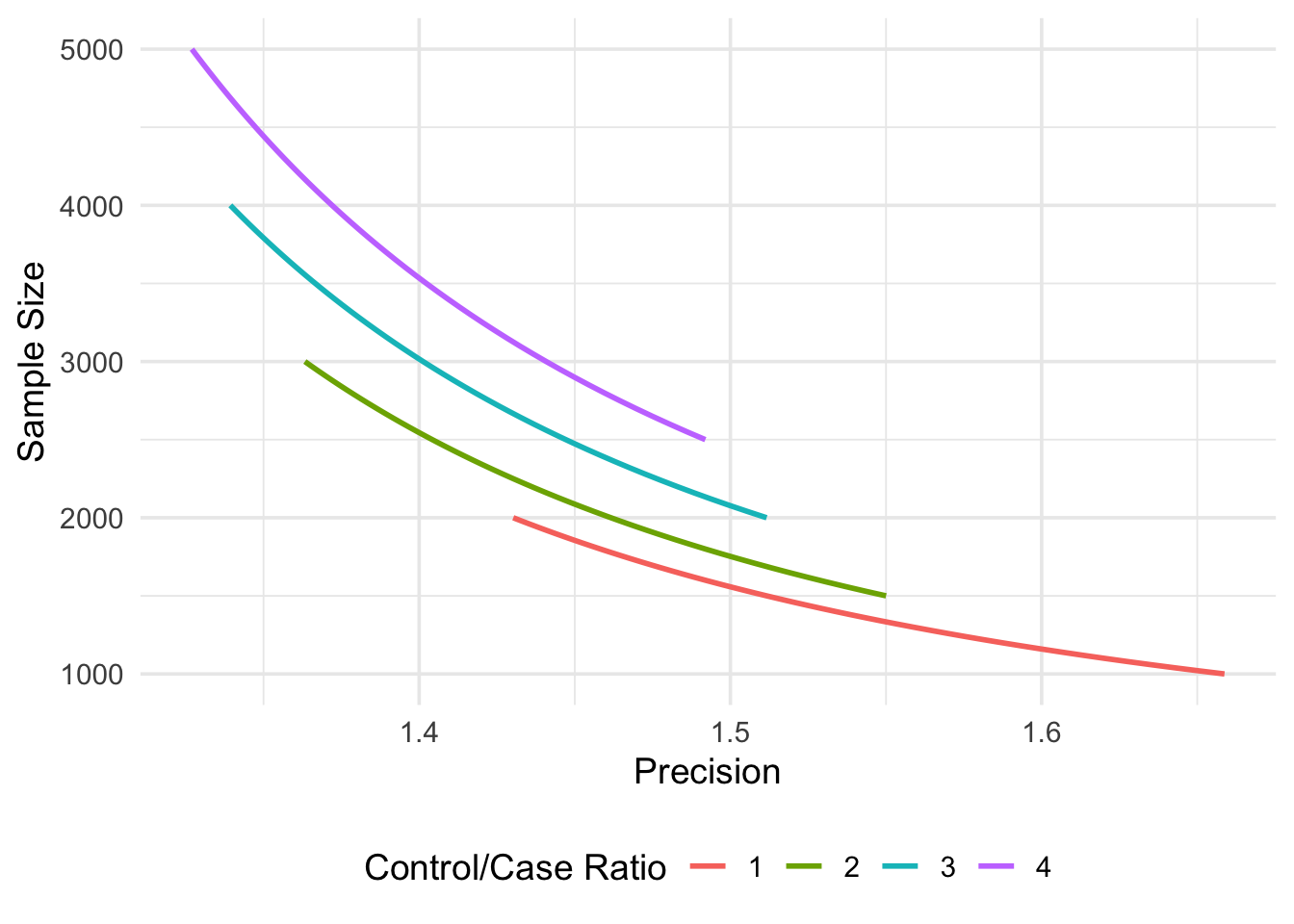# Estimating sample size for precision: precisely 0.1.0

I’m pleased to announce that precisely 0.1.0 is now on CRAN! precisely is a study planning tool to calculate sample size based on precision rather than power. Power calculations focus on whether or not an estimate will be statistically significant; calculations of precision are based on the same principles as power calculation but turn the focus to the width of the confidence interval. precisely currently supports sample size calculations for risk differences, rate differences, risk ratios, rate ratios, and odds ratios.

These tools implement the work of Rothman and Greenland. Here’s an example from their paper: We want to estimate the sample size required for a study where we are estimating a risk difference. We want the precision (here, the width of the confidence interval) to be .08. We’ll assume that the exposed participants have a disease risk of 0.4 while the unexposed have a risk of 0.3, for a risk difference of 0.1. Setting the ratio of unexposed participants to the unexposed to 3 (e.g., there are 3x more unexposed participants), we need 2097 participants to estimate a confidence interval of this precision.

``````# install.packages("precisely")
library(precisely)

n_risk_difference(
precision = .08,
exposed = .4,
unexposed = .3,
group_ratio = 3,
ci = .90
)``````
``````## # A tibble: 1 x 9
##   n_exposed n_unexposed n_total risk_difference precision exposed unexposed
##       <dbl>       <dbl>   <dbl>           <dbl>     <dbl>   <dbl>     <dbl>
## 1      524.       1573.   2097.             0.1      0.08     0.4       0.3
## # … with 2 more variables: group_ratio <dbl>, ci <dbl>``````

precisely also includes functions for making sample size figures, functions for estimating precision from the sample size, and more. See the introductory vignette to learn more about what you can do.

``````map_precisely(
precision_odds_ratio,
n_cases = seq(from = 500, to = 1000, by = 10),
exposed_cases = .6,
exposed_controls = .4,
group_ratio = 1:4
) %>%
dplyr::group_by("Control/Case Ratio" = factor(group_ratio)) %>%
plot_precision() +
theme_precisely()``````This package includes a Shiny app to help with sample size calculations, which you can start locally with `launch_precisely_app()`. You can also find an online version at malcolmbarrett.shinyapps.io/precisely.

## Making contributions to precisely

precisely has been available on GitHub for some time. If you already use precisely, there isn’t much new in the official CRAN release except some polishing and minor bug fixes. Over the past couple of years, I have received several requests for sample size calculations for different effect types and study designs. While I haven’t had time to implement these, this is an excellent opportunity to contribute to the package! Please feel free to make pull requests to the GitHub repository or suggest calculations that precisely could make in an issue (preferably with some code to do the calculation or a clear reference that explains how).##### Malcolm Barrett
###### Data Scientist

I am a data scientist, an R developer, and an epidemiologist. My work in public health has spanned on-ground clinical education and research for clinical and cohort studies. Previously, I was an intern at RStudio, and I served two years in AmeriCorps at federally-qualified health centers in Michigan and New York City.